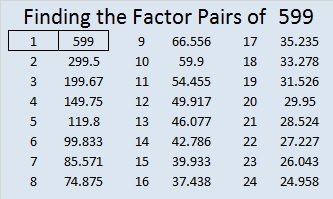# What often happens to a number like 599 when a number next to it has so many factors?

The square root of any number from 576 to 624 is between 24 and 25. That means the first number in any of their factor pairs will be 24 or less. The combined number of factor pairs for the 49 integers from 576 to 624 is 189. The number of factor pairs for any given number ranges from 1 to 12. Let’s look at the averages: The mean (189/49) is 3.857 factor pairs per number. The median is 3 factor pairs, and the mode (the number of factor pairs that occurs most often) is 4 factor pairs.What happens to a number like 599 when a number next to it has far more than the average number of factor pairs? Quite often, but not always, that number has no choice but to be a prime number.Even though 600 has only three prime factors (2, 3 and 5), it still managed to be divisible by 50% of the numbers from 1 to 24, and there just aren’t many possibilities left for the numbers immediately before or after it.

The twelve numbers less than or equal to 24 that will divide into 600 are 1, 2, 3, 4, 5, 6, 8, 10, 12, 15, 20 and 24.

Because 9, 14, 16, 18, 21, and 22 each have 2 or 3 as a prime factor, those six numbers also are not possible factors of 599 or 601.

Every whole number is divisible by 1, but besides that, there are only six numbers available as possible factors for those two numbers: 7, 11, 13, 17, 19, and 23. Since neither 599 nor 601 is divisible by any of those numbers, they turn out to be twin primes.

Usually at least one of the numbers before or after a number with far more than its fair share of factor pairs will be a prime number.

119 and 121, the numbers before and after 120 are notable exceptions. √120 ≈ 10.95441. The factors of 120 that are less than or equal to 10 are 1, 2, 3, 4, 5, 6, 8, and 10 which is 80% of the possible factors. Yet 119 managed to be divisible by 7, and 121 managed to be divisible by 11 so neither one of them is a prime number.

————————————————————————————-

If you add up the digits of 499, 589, or 598, you will get 22.

599 is the smallest whole number whose digits add up to 23. Thank you, Stetson.edu for that number fact.

• 599 is a prime number and a twin prime with 601.
• Prime factorization: 599 is prime.
• The exponent of prime number 599 is 1. Adding 1 to that exponent we get (1 + 1) = 2. Therefore 599 has exactly 2 factors.
• Factors of 599: 1, 599
• Factor pairs: 599 = 1 x 599
• 599 has no square factors that allow its square root to be simplified. √599 ≈ 24.4744765How do we know that 599 is a prime number? If 599 were not a prime number, then it would be divisible by at least one prime number less than or equal to √599 ≈ 24.5. Since 599 cannot be divided evenly by 2, 3, 5, 7, 11, 13, 17, 19, or 23, we know that 599 is a prime number.

## 4 thoughts on “What often happens to a number like 599 when a number next to it has so many factors?”

1.wbhs62

I guess I was intuitively aware of this, but it’s nice to see it argued out, and the skyscraper display is very attractive.

•ivasallay

Thank you. Many others have been intuitively aware as well. For example http://t.co/qD1MAHzysN.

2.Steve Morris

So is this a good method for finding new primes?

•ivasallay

It’s been used for centuries. Here’s some prime numbers that are next to other numbers that have a lot of factors: (2^607) – 1 and (597!!! + 1). The first one is a Mersenne prime. The largest prime number known to man is also one of those.

This site uses Akismet to reduce spam. Learn how your comment data is processed.